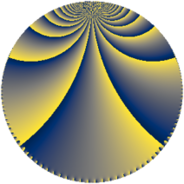# Properties

 Label 1344.4.jLevel $1344$ Weight $4$ Character orbit 1344.j Rep. character $\chi_{1344}(1247,\cdot)$ Character field $\Q$ Dimension $144$ Sturm bound $1024$

# Related objects

## Defining parameters

 Level: $$N$$ $$=$$ $$1344 = 2^{6} \cdot 3 \cdot 7$$ Weight: $$k$$ $$=$$ $$4$$ Character orbit: $$[\chi]$$ $$=$$ 1344.j (of order $$2$$ and degree $$1$$) Character conductor: $$\operatorname{cond}(\chi)$$ $$=$$ $$24$$ Character field: $$\Q$$ Sturm bound: $$1024$$

## Dimensions

The following table gives the dimensions of various subspaces of $$M_{4}(1344, [\chi])$$.

Total New Old
Modular forms 792 144 648
Cusp forms 744 144 600
Eisenstein series 48 0 48

## Trace form

 $$144q + O(q^{10})$$ $$144q + 3600q^{25} - 1392q^{33} - 7056q^{49} + 2064q^{57} - 5520q^{81} + O(q^{100})$$

## Decomposition of $$S_{4}^{\mathrm{new}}(1344, [\chi])$$ into newform subspaces

The newforms in this space have not yet been added to the LMFDB.

## Decomposition of $$S_{4}^{\mathrm{old}}(1344, [\chi])$$ into lower level spaces

$$S_{4}^{\mathrm{old}}(1344, [\chi]) \cong$$ $$S_{4}^{\mathrm{new}}(24, [\chi])$$$$^{\oplus 8}$$$$\oplus$$$$S_{4}^{\mathrm{new}}(96, [\chi])$$$$^{\oplus 4}$$$$\oplus$$$$S_{4}^{\mathrm{new}}(168, [\chi])$$$$^{\oplus 4}$$$$\oplus$$$$S_{4}^{\mathrm{new}}(192, [\chi])$$$$^{\oplus 2}$$$$\oplus$$$$S_{4}^{\mathrm{new}}(672, [\chi])$$$$^{\oplus 2}$$